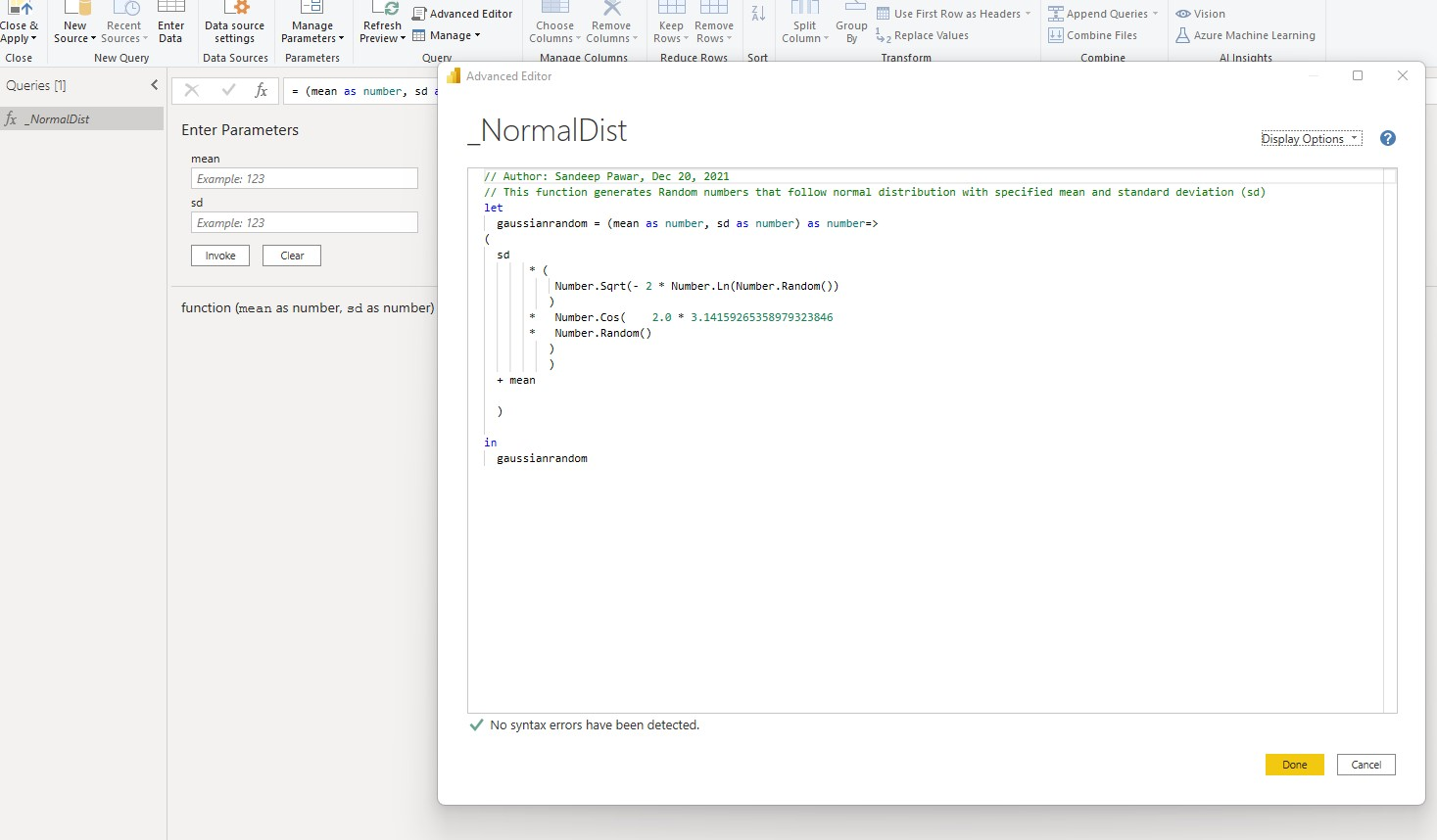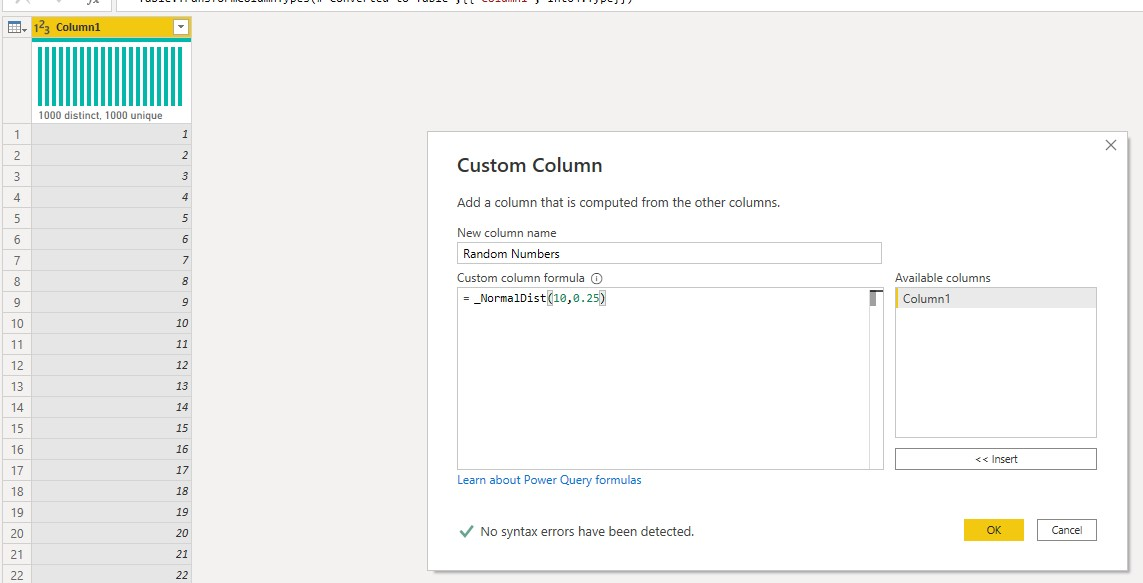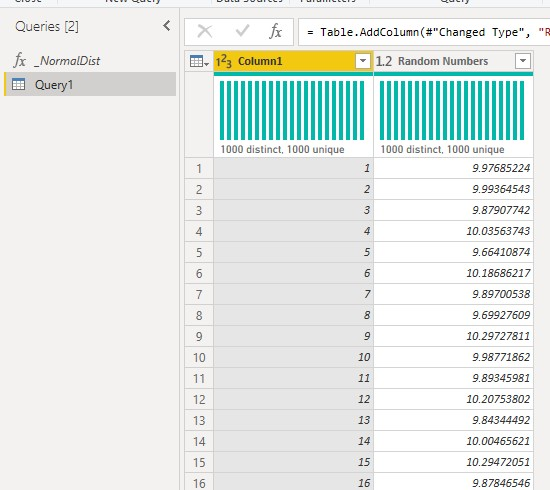## Power Query Doesn't Have NORMINV()

In Excel, if you want to generate a column with random numbers that are normally distributed, you can use the `NORMINV()` function like this. You can specify the probability (which is usually a random number drawn from uniform distribution), mean and standard deviation. While DAX has the `NORM.INV()` function, M does not. If you create simulations, what-if scenario analyses etc., more than likely you will need to generate a column with random numbers that follow the Gaussian distribution. I have written a blog post on how to generate various distributions using DAX, you can read it here.

In this blog, I will share a simple formula to generate the normally distributed random numbers using M. It uses the Box-Muller transform to generate the inverse distribution. I won't go into the theory and math, but if you are interested you can read it here.## Custom Function

```#hide-output
// Gaussian Random Number Generator with mean =mean and standard number as sd using Box-Mueller Transform
// Add an index column to the table before invoking this function.
let
gaussianrandom = (mean as number, sd as number) as number=>
(
sd
* (
Number.Sqrt(- 2 * Number.Ln(Number.Random())
)
*   Number.Cos(    2.0 * 3.14159265358979323846
*   Number.Random()
)
)
+ mean

)

in
gaussianrandom
```

## Steps

• Create a Power Query function using the formula above. In the below example, I named the function `_NormalDist`• You will need to have unique rows. If you don't, create an index column (Add Column > Index Column).
• To create a new column that follows the Gaussian distribution using the above function, go to Add Column and use the above function. In the example below, I created a new column that has mean of 10 and standard deviation of 0.25• Here is the result:Refresh the report and you will see the numbers in your table. If you see same number on all rows, just add another index column and remove it again.

`NORM.INV()` in DAX generates new numbers every time the report is refreshed. In Power Query you can disable the refresh for this table, and hence generated numbers will stay the same even after refreshing the report. If you open the PowerQuery, however, it will generate new numbers. You can use `Table.Buffer()` to freeze it but I haven't had luck with that. If you know how to do it, please let me know.

Here is the resulting distribution:

```df = pd.read_clipboard().set_index('Column1')
```
Random Numbers
Column1
1 10.104795
2 9.967639
3 10.172852
4 10.163127
5 10.043073
```sns.displot(df['Random Numbers'], rug=True, kde=True);
``````print("The mean and standard deviation of random numbers : ", round(df['Random Numbers'].mean(),2), round(df['Random Numbers'].std(),2))

```
```The mean and standard deviation of random numbers :  9.99 0.25
```

I also wrote another function to generate Traingular Distribution,which is very common to simulate risk profiles. Hope to share that soon.